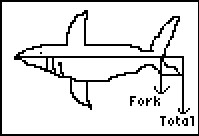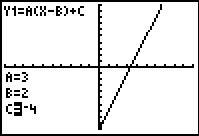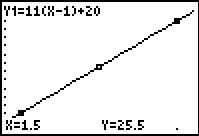••• ##### Device
• TI-83 Plus Family
• TI-84 Plus
• TI-84 Plus Silver Edition
• ##### Report an Issue

Algebra I: Shark Attack
by Texas Instruments - Bell Ringer Lesson#### Overview

Students use the Transformation Graphing application to separate what effect each change in the Point-Slope equation has on the graph.

#### Key Steps

•This activity introduces the students to both forms of the Point-Slope form of an equation. Students will use the Transformation Graphing App to experiment with each part of the equation one at a time in order to understand the changes that take place as each number changes.

•Problem 2 introduces a contextual problem so that students now think about the ordered pairs as data points, and the slope as a rate of change. In this case, the growth of a child is used. Students will calculate the slope, write the equation in point-slope form, and then graph the equation with the ordered pairs. From the graph, they will be able to make “predictions.”

•Students will repeat the same process for Problem 3 where they will investigate the total body length and the fork length of a shark.